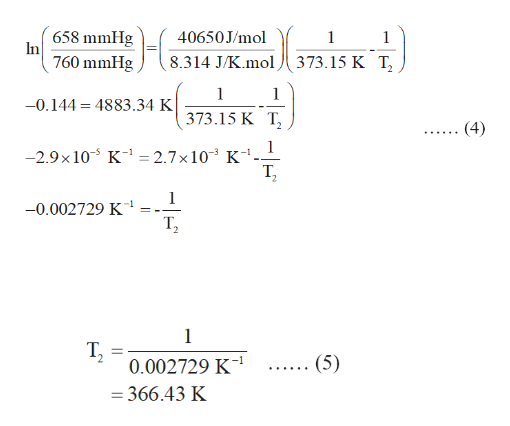# 1. A pipet delivers 1.03 g  of water at 18 oC . What volume (in mL) does the pipet deliver?Use the table in your lab manual to assist you with determining the density of water at a specific temperature.Answer: ____ mL2. Determine the boiling point of water at 658 mmHg .Answer: ____ degrees celsius

Question
14 views
1. A pipet delivers 1.03 g  of water at 18 oC . What volume (in mL) does the pipet deliver?
Use the table in your lab manual to assist you with determining the density of water at a specific temperature.
2. Determine the boiling point of water at 658 mmHg .
check_circle

Step 1

Density of a substance is the ratio of its mass and volume. It is expressed in equation (1) in which d is the density, m is the mass and V is the volume.

The expression for Clausius Clapeyron equation is expressed in equation (2) in which P1 and P2 are two different pressures, T1 and T2 are two different temperatures, ∆Hvap is the enthalpy of vaporization and R is the gas constant.

Step 2

(1)

The density of water at 18 degrees Celsius is 0.998595 g/mL and the mass of water is 1.03 g. Use the equation (1) to determine the volume of water as shown in equation (3) .

Step 3

(2)

The enthalpy of vaporization of water (∆Hvap ) is 40650 J/mol. Water boils at 373.15 K. Use the equation (2) to determine the boiling point of water and substitute 760 mmHg for P1, 658 mmHg for P2, 373.15 K for T...help_outlineImage Transcriptionclose658 mmHg In 760 mmHg 40650 J/mol 1 1 8.314 J/K.mol 373.15 K T 1 1 -0.144 4883.34 K 373.15 K T (4) 1 -2.9x 10 K - 2.7x 103 K Т, -1 1 1 -0.002729 K Т, 1 Т, 0.002729 K1 (5) -366.43 K fullscreen

### Want to see the full answer?

See Solution

#### Want to see this answer and more?

Solutions are written by subject experts who are available 24/7. Questions are typically answered within 1 hour.*

See Solution
*Response times may vary by subject and question.
Tagged in

### Chemistry